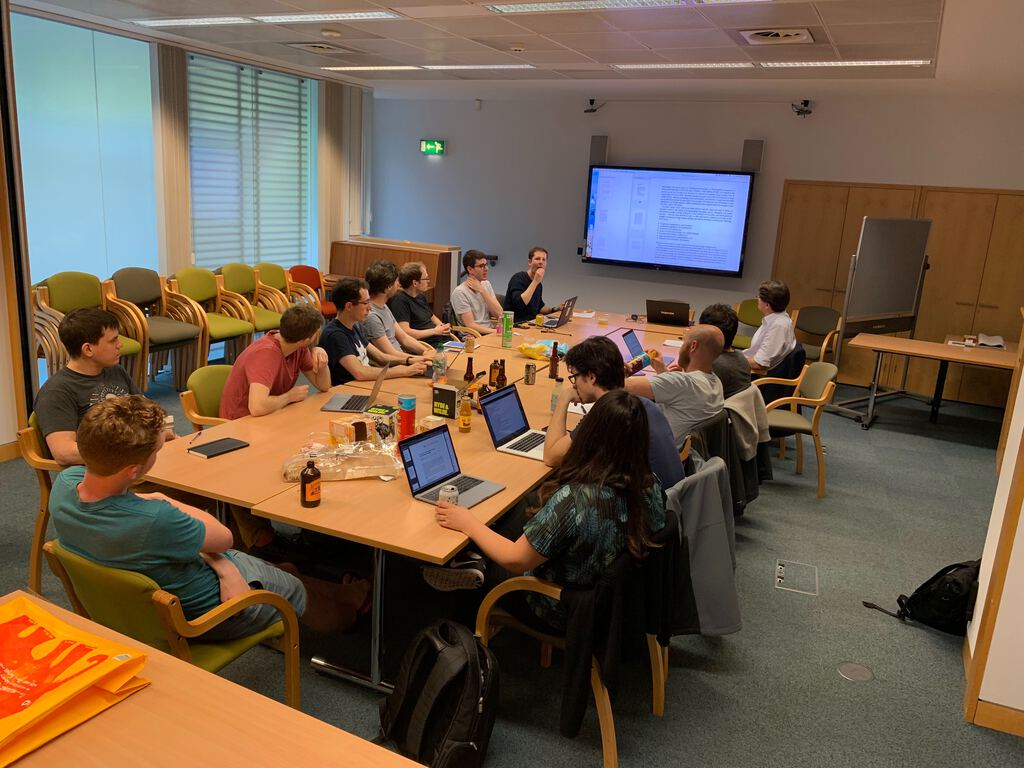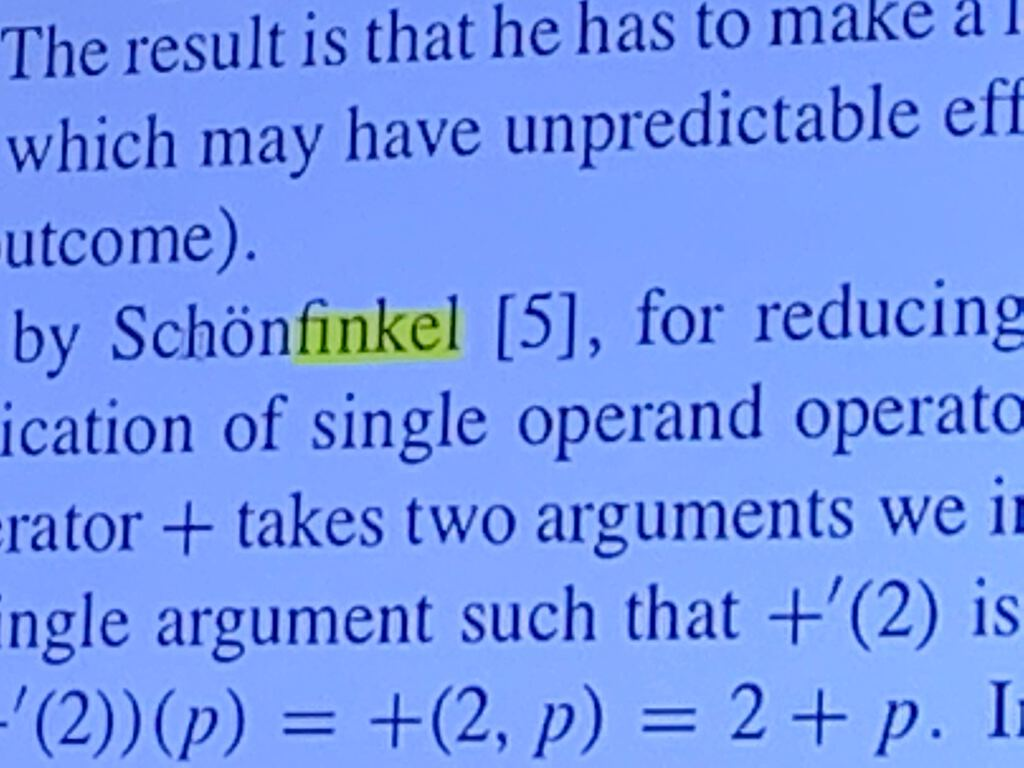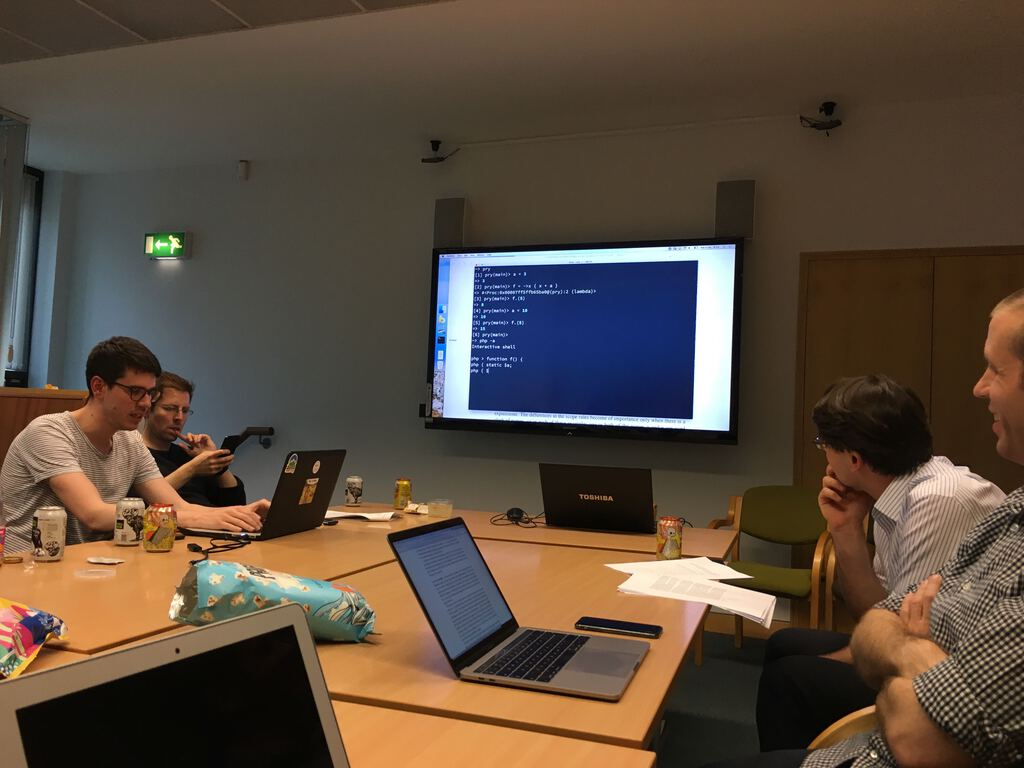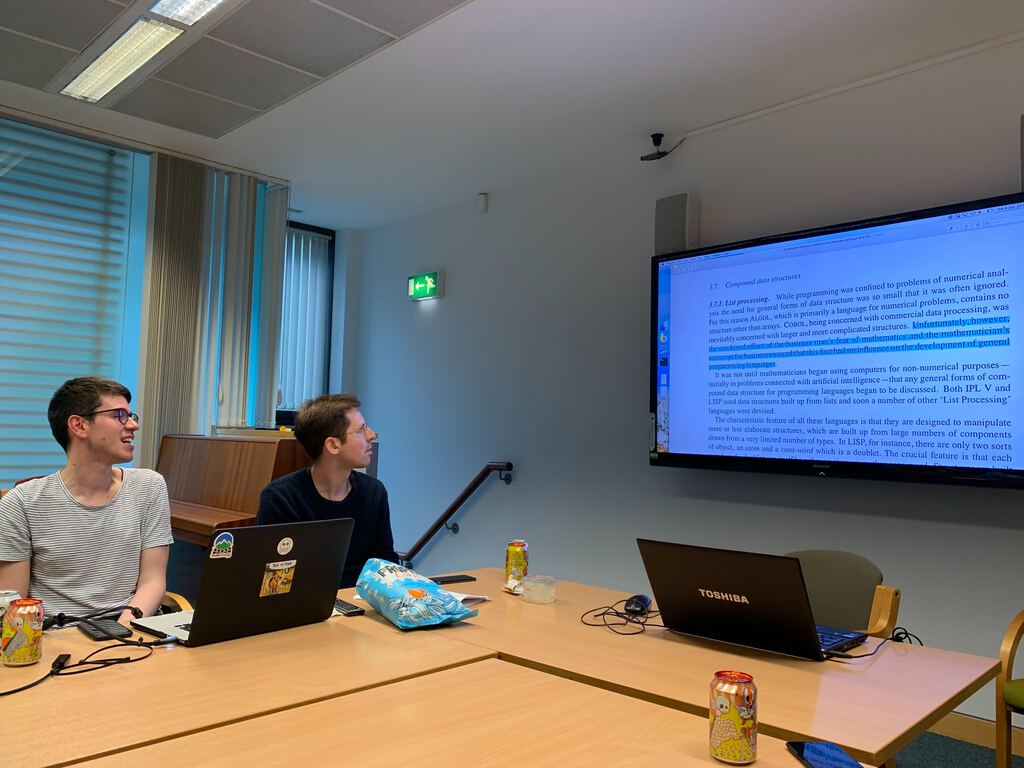10TPEPSR Fundamental Concepts in Programming Languages

The meetingPre-meeting notes

• Notes for a lecture series given in 1967, one person's opinions on programming language theory at the time
• The notes were circulated for a long time but only finally published in 2000
• 1: Preliminary section, why studying programming languages is important
• 1.1: Need formal jargon because it's so vague and we need to communicate effectively, e.g. what does "name" mean?
• 1.2: Philosophical outlook: at this point in time, CS are still all mathematicians, constructivist vs classical mathematics
• The important thing to study with programming languages is semantics (what can the language do), syntax isn't really important
• Compares the state of programming language theory with Calculus when it was called the "Method of Fluxions" before they figured out differentials
• Be very careful about what we formalise: "no axiomatisation without insight" but, on the flip side, avoid "perpetual vagueness"
• Section 2: Core PL concepts, code examples are in CPL
• 2.1 Assignment was totally novel at this point: unlike mathematics, computers have memory you can store things in
``````x = 3
x = y + 1
x = x + 1
``````
• Complex, conditional assignment
``````a > b ? j : k = i
``````
• General form:
``````left = right
``````
• 2.2 L-values and R-values
• L-values = location (not just a pointer)
• R-values = values
• 2.3 Definitions
• Declare a new L-value with an R-value: `p = 3.5`
• Aliases (not commonly implemented): assigning to `x` would set `M[2,2]`
``````let x ≃ M[2,2]
``````
• 2.4: "Name", what we'd now call identifiers; Algol's "call by name" confuses things
• 2.5: Numerals are "names of numbers", e.g. numbers written with different bases, e.g. `0253`
• 2.6: Conceptual model of L-values and R-values with names, numerals, numbers and vectors
• Names have L-values that point to an R-value
• Numerals don't have L-values but point directly to an R-value
• Vector have L-values that point to other L-values (which then point to R-values)
• Section 3: Conceptual constructs
• 3.1: Expressions are pure, e.g.
``````1
1+ 1
sin(1 + x + y / x^2)
``````
• Commands:
``````x = 1
y = 1 + 1
z = sin(1 + x + y / x^2)
``````
• Expressions involve only R-values (like maths)
• Commands involve at least one L-value
• Keep expressions and commands separate
• Power of programming language comes from how complex expressions can be
• 3.2: How expressions work
• Every expression has a value, let's focus on their R-values (ignore the L-values)
• Referential transparency: the only thing you need to know about expressions are their values, you don't need to care about their form
``````sin(6)
sin(1 + 5)
sin(30 / 5)
``````
• Think only about calculating the value of a single expression at a time
• All expressions exist in reference to some environment which provides the meaning of all variables
``````a + 3/a where a = 2 + 3/7
a + b - 3/a where a = b + 2/b
``````
• `a` is bound to a value, `b` is free as its value is not bound and must still be looked up in the environment
• 3.2.3: Application structure, S-expressions
``````a + b == +(a, b)
``````
• No precedence rules, application order is explicit
• Operators can be expressions too
``````D(sin) = cos
(D(sin))(*(3, a)) == cos(3 * a)
``````
• 3.2.4: Evaluation
1. Evaluate the operator and the operands in any order
2. Apply operator to the operands
• Partial order on expressions: which expressions need to be evaluated in which order
• Is an order of evaluation defined? Could we evaluate operands in parallel?
• 3.2.4: Conditional expressions
``````x == 0 ? 0 : 1/x
``````
• We don't want to evaluate all the operands here
• Do it with lambda expressions instead
• 3.3: Order of evaluation is very important with commands
• 3.3.1: Variables (in maths, they do not vary)
• Cost of introducing commands (changing R-values in L-values), side effects are hard to reason about
• 3.3.2: Abstract store: σ-notation, basically a symbol table of L-values to R-values, σ operations return a new symbol table
• 3.4: Functions and routines: functions are portable expressions; routines are portable commands
``````f(x) = 5 * x^2 + 3 * x + 2 / x^3
f = -> x { 5 * x^2 + 3 * x + 2 / x^3 }
``````
• 3.4.2: Parameter calling modes: do we pass bound variables as L-values or R-values as arguments?
• Fortran pass everything by R-value, ALGOL pass by L-value but ALGOL60 had "call by name" (thunks)
• 3.4.3: Free variables
``````f(x) = x + a

# Free variable captured by R-value
a = 3
f(x) = x + a
# f(5) => 8
a = 10
# f(5) = 8

# By L-value
a = 3
f(x) = x + a
# f(5) => 8
a = 10
# f(5) = 15
``````
• 3.4.4: Own variable (like static variables in C/PHP)
• 3.4.5: Functions and routines: as different as expressions and commands, side-effect-free or not
• Try to avoid breaking referential transparency
• 3.4.6: deal with with side effects not through functions/routines but by freezing the data they operate on
• Constants: property of an L-value where its R-value cannot be changed
• 3.4.7: fixed and free functions
• Fixed: all variables are bound
• Free: not all variables ar ebound
• Fixed: it's clear what it will do, can be compiled separately
• 3.4.8: Fixed functions can generate free functions at run-time
• 3.5: First-class object distinction
• 3.5.1: First and second class objects
``````(x > 1 ? a : b) + 6
(x > 1 ? sin : cos)(6)
``````
• Rationale that functions aren't first-class because mathematics assumes functions have simple names
• 3.5.2: What is the R-value of a function? A closure with how to evaluate the function and its environment
• 3.6: Types and polymorphism
• Types are the representation and the range
• Operations that are ambiguous unless you know the types of the arguments are polymorphic
• Are types an attribute of the L-value or the R-value?
• Manifest: types are an attribute of L-values
• Latent: types are an attribute of R-values
• 3.6.2: When can we figure out the types? At compile time or run time?
• Compile type: manifest
• Run time: latent
• 3.6.3: if only R-values have types (as in dynamic languages)
• 3.6.4: ad hoc polymorphism there is no single way to determine the type of result from the type of the arguments
• Parametric polymorphism where we know the type of the argument and the result
• 3.6.5: Types of functions include types and modes of calling its parameters and the types of its results
• Variadic functions are a form of polymorphic functions
• 3.7.1: once people used computers for non-numeric purposes then they discovered data structures such as arrays
• 3.7.2: build types out of nodes (new entities that have no uncertainty) and elements (new entities that have uncertainty, e.g. maybe it's a scalar or maybe I'm a doublet)
• 3.7.3: we need to know the L- and R-values to understand assignment with data structures
• 3.7.4: pointer bit hacks
• 3.7.6: pointers, an R-value can reference a location, two primitive operations: Follow and Pointer
• Follow: given a pointer, where is its R-value?
• Pointer: given an L-value, give an R-value pointing to it
• 3.7.7: other data structures, e.g. List, Ntuple, Set, Bag
• Section 4: Miscellaneous topics
• Load-Update Pairs: formal exposition of L-values
• Macrogenerators: programs are just strings of symbols (nothing more), ways of manipulating the symbols (basically macros in Lisp, Rust, etc.)

Thanks

Thanks to [@tomstuart], [@urbanautomaton] and FutureLearn for hosting the meeting and providing drinks and bread and thanks to all who brought dips and snacks.

Clone this wiki locally
You can’t perform that action at this time.